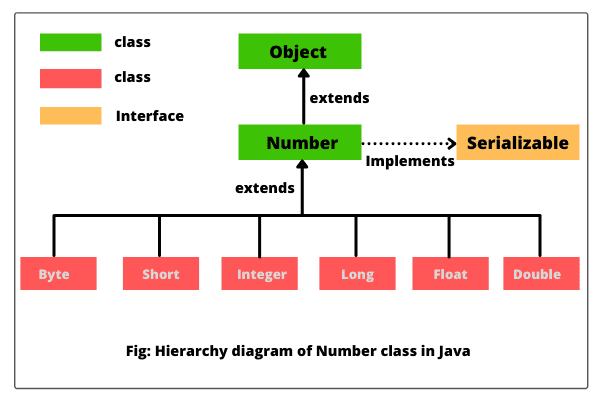# Java Number Class | Methods, Example

Number class in Java is an abstract class that is the superclass of Byte, Short, Integer, Long, Float, and Double classes.

The Byte, Short, Integer, Long, Float, and Double classes are the most commonly used wrapper classes that represent numeric values.

All the numeric type wrappers inherit from abstract class Number.

Java Number class and its subclasses are present in java.lang package. It was added in Java 1.0 version.

## Number class declaration

The abstract Number class extends Object class and implements Serializable interface. The general declaration of Number class is as follows:

```public abstract class Number
extends Object
implements Serializable```

The classes such as AtomicInteger, AtomicLong, BigDecimal, BigInteger, DoubleAccumulator, DoubleAdder, LongAccumulator, and LongAdder also implement Number class.

The hierarchy diagram of Number class in Java is shown in the below figure.## Number class methods in Java

Number class defines some useful methods that return the value of an object in each of the different number formats. These methods are as follows:

1. byte byteValue(): This method returns the value of an object as a byte which may involve rounding or truncation. In other words, it converts the calling object into byte value. The calling object may be an object of Byte, Short, Integer, Long, Float, or Double class.

2. short shortValue(): This method returns the value of an object as a short which may involve rounding or truncation. In simple words, it converts the calling object into short value.

3. abstract int intValue(): It returns the value of an object as a short which may involve rounding or truncation. That is, it converts the calling object into int value.

4. abstract long longValue(): It returns the value of an object as long which may involve rounding or truncation. It is used to convert the calling object into long value.

5. abstract float floatValue(): This method returns the value of an object as float which may involve rounding.

6. abstract double doubleValue(): This method returns the value of an object as long which may involve rounding.

All these methods provided by abstract Number class in java are available to Byte, Short, Integer, Long, Float, and Double classes.

## Example Program based on Number class in Java

1. Let’s take an example program where we will find out the integer range constants, floating-point range constants, and some other useful range constants. Look at the source code.

Program code 1:

```public class Test
{
public static void main(String[] args)
{
// Integer range constants:
System.out.println("Range of Byte: "+Byte.MIN_VALUE+ " to "+ Byte.MAX_VALUE);
System.out.println("Range of Short: " +Short.MIN_VALUE+ " to" + Short.MAX_VALUE);

System.out.println("Range of Integer: " +Integer.MIN_VALUE+ " to" + Integer.MAX_VALUE);
System.out.println("Range of Long: " +Long.MIN_VALUE+ " to" + Long.MAX_VALUE);

// Floating-point range constants.
System.out.println("Range of Float: " +Float.MIN_VALUE+ " to" + Float.MAX_VALUE );
System.out.println("Range of Double: " +Double.MIN_VALUE+ " to" + Double.MAX_VALUE );

// Other useful constants.
System.out.println("Math.PI: " +Math.PI);
System.out.println("Math.E: " +Math.E);
}
}```
```Output:
Range of Byte: -128 to 127
Range of Short: -32768 to32767
Range of Integer: -2147483648 to2147483647
Range of Long: -9223372036854775808 to9223372036854775807
Range of Float: 1.4E-45 to3.4028235E38
Range of Double: 4.9E-324 to1.7976931348623157E308
Math.PI: 3.141592653589793
Math.E: 2.718281828459045```

2. Let’s take an example program where we will get the value of object Double after converting it into byte, short, int, long, float, and double.

Program code 2:

```public class Test
{
public static void main(String[] args)
{
// Creating a Double Class object having a value "25.10"
Double d = new Double("25.10");

// Converting this Double(Number) object to different primitive data type values.
byte b = d.byteValue();
short s = d.shortValue();

int i = d.intValue();
long l = d.longValue();

float f = d.floatValue();
double d1 = d.doubleValue();

System.out.println("byteValue: " + b);
System.out.println("shortValue: " + s);

System.out.println("intValue: " + i);
System.out.println("longValue: " + l);

System.out.println("floatValue: " + f);
System.out.println("doubleValue: " + d1);
}
}```
```Output:
byteValue: 25
shortValue: 25
intValue: 25
longValue: 25
floatValue: 25.1
doubleValue: 25.1```

Hope that this tutorial has covered important points related to Java Number class with example programs. I hope that you will have understood this simple topic in Java programming language.

Please inform our team via email if you find anything incorrect in this tutorial. Your email will be precious to us.